# NAS with RL

2017-ICLR-Neural Architecture Search with Reinforcement Learning

Quoc V . Le etc
GitHub： stars
Citation：1499

## Abstract

we use a recurrent network to generate the model descriptions of neural networks and train this RNN with reinforcement learning to maximize the expected accuracy of the generated architectures on a validation set.

## Motivation

Along with this success is a paradigm shift from feature designing to architecture
designing,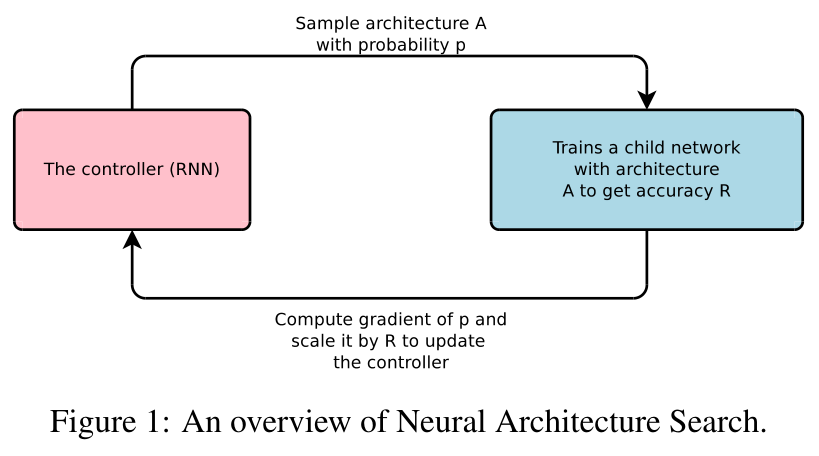This paper presents Neural Architecture Search, a gradient-based method for finding good architectures (see Figure 1) .

Our work is based on the observation that the structure and connectivity of a neural network can be typically specified by a variable-length string.

It is therefore possible to use a recurrent network – the controller – to generate such string.

Training the network specified by the string – the “child network” – on the real data will result in an accuracy on a validation set.

Using this accuracy as the reward signal, we can compute the policy gradient to update the controller.

As a result, in the next iteration, the controller will give higher probabilities to architectures that receive high accuracies. In other words, the controller will learn to improve its search over time.

## Method

### 3.1 GENERATE MODEL DESCRIPTIONS WITH A CONTROLLER RECURRENT NEURAL NETWORK

Let’s suppose we would like to predict feedforward neural networks with only convolutional layers, we can use the controller to generate their hyperparameters as a sequence of tokens: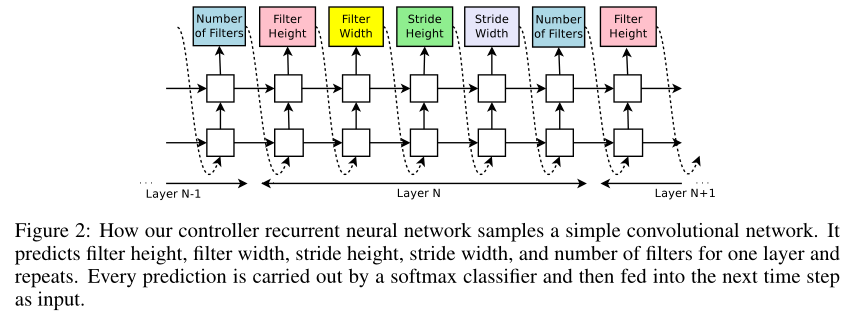In our experiments, the process of generating an architecture stops if the number of layers exceeds a certain value.

This value follows a schedule where we increase it as training progresses.

Once the controller RNN finishes generating an architecture, a neural network with this architecture is built and trained.

At convergence, the accuracy of the network on a held-out validation set is recorded.
（子网络训练**）收敛时，记录验证集上的准确率。

The parameters of the controller RNN, θc, are then optimized in order to maximize the expected validation accuracy of the proposed architectures.

In the next section, we will describe a policy gradient method which we use to update parameters θc so that the controller RNN generates better architectures over time.

### 3.2 Training with Reinforce

The list of tokens that the controller predicts can be viewed as a list of actions $$a_{1:T}$$ to design an architecture for a child network.

At convergence, this child network will achieve an accuracy R on a held-out dataset.

We can use this accuracy R as the reward signal and use reinforcement learning to train the controller.

More concretely, to find the optimal architecture, we ask our controller to
maximize its expected reward, represented by $$J(θ_c)$$:

$$J\left(\theta_{c}\right)=E_{P\left(a_{1: T} ; \theta_{c}\right)}[R]$$.
⭐️ **如何计算R的期望？$$P\left(a_{1: T} ; \theta_{c}\right)$$,是什么？

Since there ward signal R is non-differentiable, we need to use a policy gradient method to iteratively update $$θ_c$$.

$$\nabla_{\theta_{c}} J\left(\theta_{c}\right)=\sum_{t=1}^{T} E_{P\left(a_{1: T} ; \theta_{c}\right)}\left[\nabla_{\theta_{c}} \log P\left(a_{t} | a_{(t-1): 1} ; \theta_{c}\right) R\right]$$.
⭐️ **$$P\left(a_{t} | a_{(t-1): 1} ; \theta_{c}\right)$$.是什么？$$\sum_{t=1}^{T}$$.又是什么？

An empirical approximation of the above quantity is:

$$\frac{1}{m} \sum_{k=1}^{m} \sum_{t=1}^{T} \nabla_{\theta_{c}} \log P\left(a_{t} | a_{(t-1): 1} ; \theta_{c}\right) R_{k}$$.
⭐️ 怎么近似的？

Where m is the number of different architectures that the controller samples in one batch and T is the number of hyperparameters our controller has to predict to design a neural network architecture.

The validation accuracy that the k-th neural network architecture achieves after being trained on a training dataset is $$R_k$$.
$$R_k$$是第k个结构的训练精度

The above update is an unbiased estimate for our gradient, but has a very high variance. In order to reduce the variance of this estimate we employ a baseline function:

$$\frac{1}{m} \sum_{k=1}^{m} \sum_{t=1}^{T} \nabla_{\theta_{c}} \log P\left(a_{t} | a_{(t-1): 1} ; \theta_{c}\right)\left(R_{k}-b\right)$$

As long as the baseline function b does not depend on the on the current action, then this is still an unbiased gradient estimate.

In this work, our baseline b is an exponential moving average of the previous architecture accuracies.

In Neural Architecture Search, each gradient update to the controller parameters $$θ_c$$ corresponds to training one child net-work to convergence.
⭐️ 每次训练一个子网络到收敛时才更新控制器RNN的梯度？

As training a child network can take hours, we use distributed training and asynchronous parameter updates in order to speed up the learning process of the controller (Dean et al., 2012).

We use a parameter-server scheme where we have a parameter server of S shards, that store the shared parameters for K controller replicas.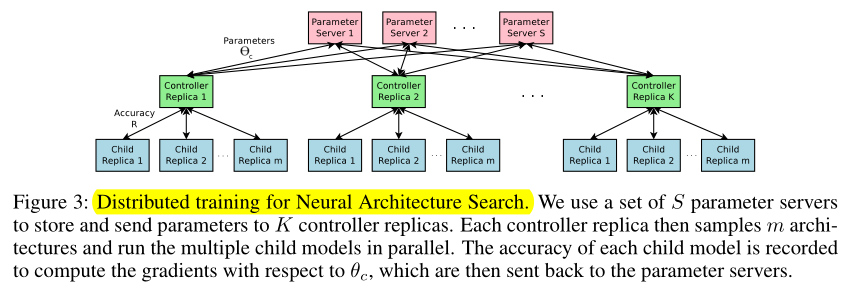### 3.3 Increase Architecture Complexity Skip Connections and Other Layer Types

In Section 3.1, the search space does not have skip connections, or branching layers used in modern architectures such as GoogleNet (Szegedy et al., 2015), and Residual Net (He et al., 2016a).

In this section we introduce a method that allows our controller to propose skip connections or branching layers, thereby widening the search space.

To enable the controller to predict such connections, we use a set-selection type attention (Neelakan-tan et al., 2015) which was built upon the attention mechanism (Bahdanau et al., 2015; Vinyals et al., 2015).

At layer N, we add an anchor point which has N − 1 content-based sigmoids to indicate the previous layers that need to be connected.

Each sigmoid is a function of the current hiddenstate of the controller and the previous hiddenstates of the previous N − 1 anchor points:

$$\mathrm{P}(\text { Layer } \mathrm{j} \text { is an input to layer } \mathrm{i})=\operatorname{sigmoid}\left(v^{\mathrm{T}} \tanh \left(W_{\text {prev}} * h_{j}+W_{\text {curr}} * h_{i}\right)\right)$$

where $$h_j$$ represents the hiddenstate of the controller at anchor point for the j-th layer, where j ranges from 0 to N − 1.

We then sample from these sigmoids to decide what previous layers to be used as inputs to the current layer.

The matrices $$W_{prev}$$, $$W_{currand}$$ ,$$v$$ are trainable parameters.

As these connections are also definedby probability distributions, the REINFORCE method still applies without any significant modifications.

Figure 4 shows how the controller uses skip connections to decide what layers it wants as inputs to the current layer.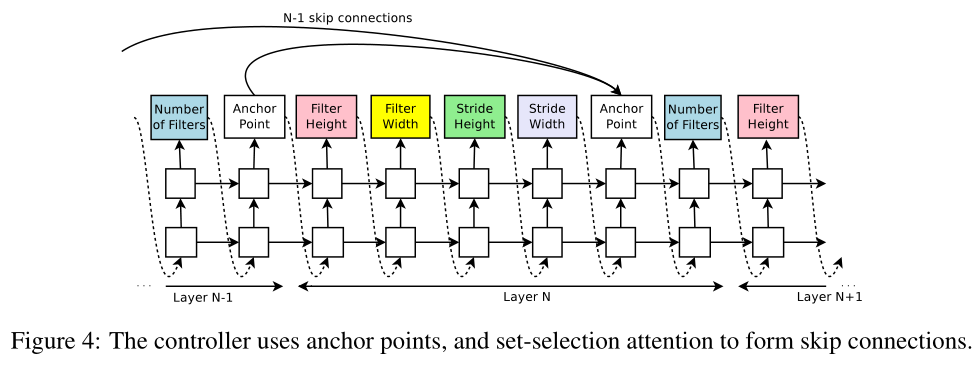In our framework, if one layer has many input layers then all input layers are concatenated in the depth dimension.

Skip connections can cause “compilation failures” where one layer is not compatible with another layer, or one layer may not have any input or output. To circumvent these issues, we employ three simple techniques.
skip connections会导致concatenated失败，比如不同层的output维度不同、一个层没有input或没有output，为了解决这个问题，我们使用了3个技术

First, if a layer is not connected to any input layer then the image is used as the input layer.

Second, at the final layer we take all layer outputs that have not been connected and concatenate them before sending this final hidden state to the classifier.

Lastly, if input layers to be concatenated have different sizes, we pad the small layers with zeros so that the concatenated layers have the same sizes.

Finally, in Section 3.1, we do not predict the learning rate and we also assume that the architectures consist of only convolutional layers, which is also quite restrictive.

It is possible to add the learning rate as one of the predictions.

Additionally, it is also possible to predict pooling, local contrast normalization (Jarrett et al., 2009; Krizhevsky et al., 2012), and batchnorm (Ioffe & Szegedy, 2015) in the architectures.

To be able to add more types of layers, we need to add an additional step in the controller RNN to predict the layer type, then other hyperparameters associated with it.

## Experiments

We apply our method to an image classification task with CIFAR-10

On CIFAR-10, our goal is to find a good convolutional architecture

. On each dataset, we have a separate held-out validation dataset to compute the reward signal.

The reported performance on the test set is computed only once for the network that achieves the best result on the held-out validation dataset.

Search space: Our search space consists of convolutional architectures, with rectified linear units(ReLU) as non-linearities (Nair & Hinton, 2010), batch normalization (Ioffe & Szegedy, 2015) and skip connections between layers (Section 3.3).

For every convolutional layer, the controller RNN has to select a filter height in [1, 3, 5, 7], a filter width in [1, 3, 5, 7], and a number of filters in [24, 36, 48, 64]. For strides, we perform two sets of experiments, one where we fix the strides to be 1, and one where we allow the controller to predict the strides in [1, 2, 3].

Training details: The controller RNN is a two-layer LSTM with 35 hidden units on each layer. It is trained with the ADAM optimizer (Kingma & Ba, 2015) with a learning rate of 0.0006. The weights of the controller are initialized uniformly between -0.08 and 0.08.

trained on 800 GPUs concurrently at any time.

Once the controller RNN samples an architecture, a child model is constructed and trained for 50 epochs.

The reward used for updating the controller is the maximum validation accuracy of the last 5 epochs cubed.

The validation set has 5,000 examples randomly sampled from the training set, the remaining 45,000 examples are used for training.

We use the Momentum Optimizer with a learning rate of 0.1, weight decay of 1e-4, momentum of 0.9 and used Nesterov Momentum

During the training of the controller, we use a schedule of increasing number of layers in the child networks as training progresses.

On CIFAR-10, we ask the controller to increase the depth by 2 for the child models every 1,600 samples, starting at 6 layers.

Results: After the controller trains 12,800 architectures, we find the architecture that achieves the best validation accuracy.

We then run a small grid search over learning rate, weight decay, batchnorm epsilon and what epoch to decay the learning rate.

The best model from this grid search is then run until convergence and we then compute the test accuracy of such model and summarize the results in Table 1.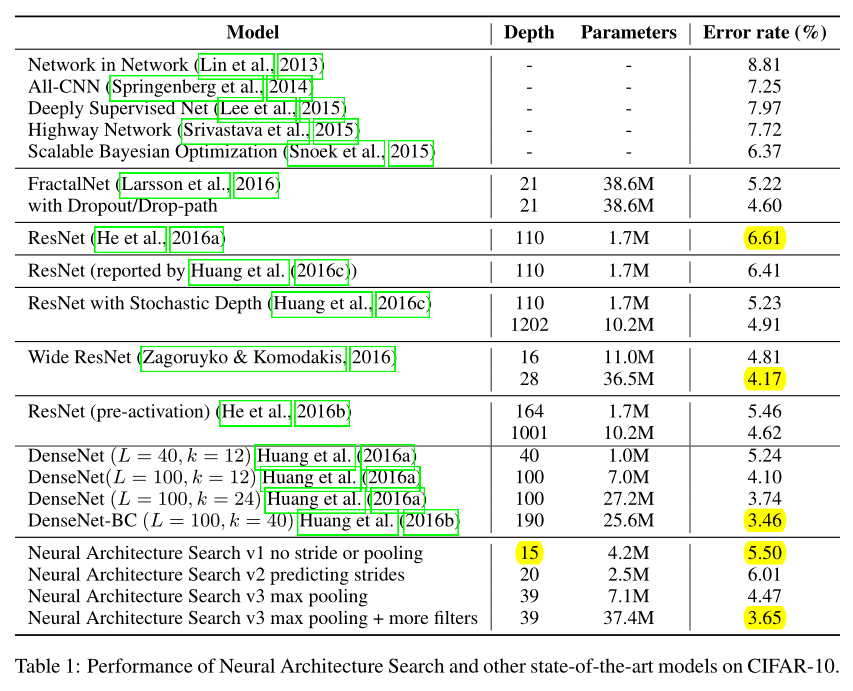First, if we ask the controller to not predict stride or pooling, it can design a 15-layer architecture that achieves 5.50% error rate on the test set.

This architecture has a good balance between accuracy and depth. In fact, it is the shallowest and perhaps the most inexpensive architecture among the top performing networks in this table.

This architecture is shown in Appendix A, Figure 7.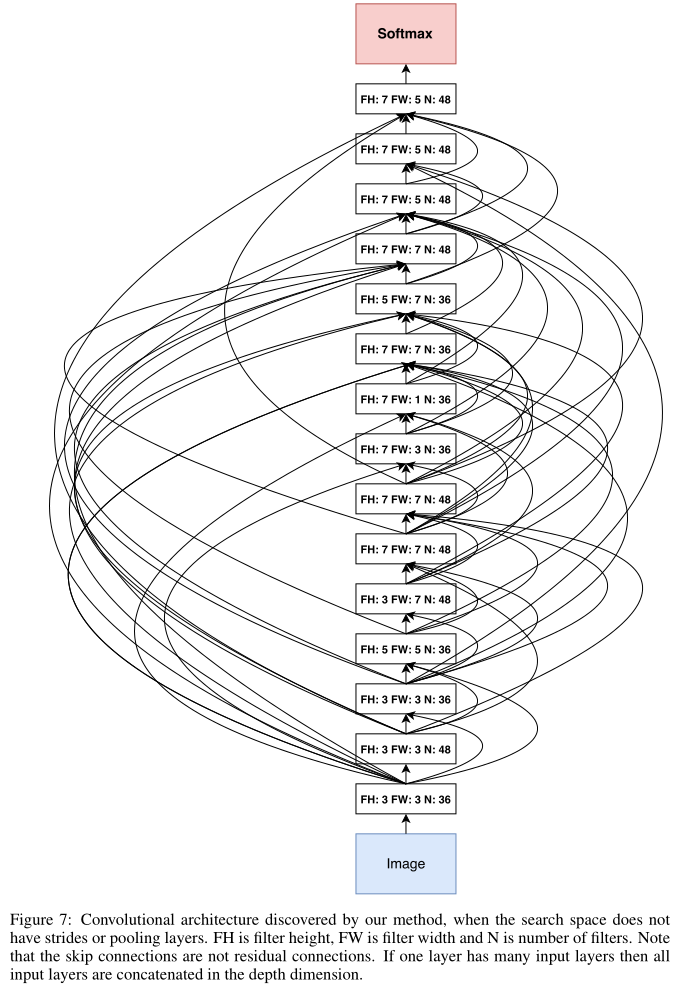A notable feature of this architecture is that it has many rectangular filters and it prefers larger filters at the top layers. Like residual networks (He et al., 2016a), the architecture also has many one-step skip connections.

This architecture is a local optimum in the sense that if we perturb it, its performance becomes worse.

In the second set of experiments, we ask the controller to predict strides in addition to other hyperparameters.

In this case, it finds a 20-layer architecture that achieves 6.01% error rate on the test set, which is not much worse than the first set of experiments.

Finally, if we allow the controller to include 2 pooling layers at layer 13 and layer 24 of the architectures, the controller can design a 39-layer network that achieves 4.47% which is very close to the best human-invented architecture that achieves 3.74%.

To limit the search space complexity we have our model predict 13 layers where each layer prediction is a fully connected block of 3 layers.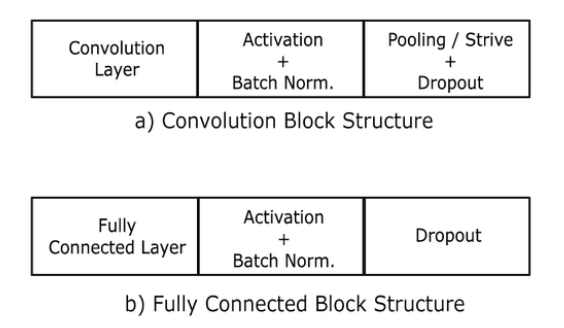Additionally, we change the number of filters our model can predict from [24, 36, 48, 64] to [6, 12, 24, 36].
Our result can be improved to 3.65% by adding 40 more filters to each layer of our architecture.
Additionally this model with 40 filters added is 1.05x as fast as the DenseNet model that achieves 3.74%, while having better performance.
The DenseNet model that achieves 3.46% error rate (Huang et al., 2016b) uses 1x1 convolutions to reduce its total number of parameters, which we did not do, so it is not an exact comparison.